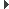ScummVM
Common::Point Struct Reference

Simple class for handling both 2D position and size. More...

`#include <rect.h>`Inheritance diagram for Common::Point:

## Public Member Functions

Point ()

Point (int16 x1, int16 y1)

bool operator== (const Point &p) const

bool operator!= (const Point &p) const

Point operator+ (const Point &delta) const

Point operator- (const Point &delta) const

void operator+= (const Point &delta)

void operator-= (const Point &delta)

uint sqrDist (const Point &p) const
Return the square of the distance between this point and the point p. More...

## Public Attributes

int16 x
The horizontal part of the point. More...

int16 y
The vertical part of the point. More...

## Detailed Description

Simple class for handling both 2D position and size.

Definition at line 37 of file rect.h.

## ◆ Point() [1/2]

 Common::Point::Point ( )
inline

Definition at line 41 of file rect.h.

## ◆ Point() [2/2]

 Common::Point::Point ( int16 x1, int16 y1 )
inline

Definition at line 42 of file rect.h.

## ◆ operator!=()

 bool Common::Point::operator!= ( const Point & p ) const
inline

Definition at line 44 of file rect.h.

## ◆ operator+()

 Point Common::Point::operator+ ( const Point & delta ) const
inline

Definition at line 45 of file rect.h.

## ◆ operator+=()

 void Common::Point::operator+= ( const Point & delta )
inline

Definition at line 48 of file rect.h.

## ◆ operator-()

 Point Common::Point::operator- ( const Point & delta ) const
inline

Definition at line 46 of file rect.h.

## ◆ operator-=()

 void Common::Point::operator-= ( const Point & delta )
inline

Definition at line 53 of file rect.h.

## ◆ operator==()

 bool Common::Point::operator== ( const Point & p ) const
inline

Definition at line 43 of file rect.h.

## ◆ sqrDist()

 uint Common::Point::sqrDist ( const Point & p ) const
inline

Return the square of the distance between this point and the point p.

Parameters
 p the other point
Returns
the distance between this and p

Definition at line 64 of file rect.h.

## ◆ x

 int16 Common::Point::x

The horizontal part of the point.

Definition at line 38 of file rect.h.

## ◆ y

 int16 Common::Point::y

The vertical part of the point.

Definition at line 39 of file rect.h.

The documentation for this struct was generated from the following file: